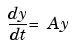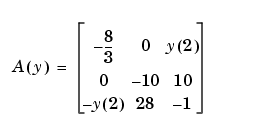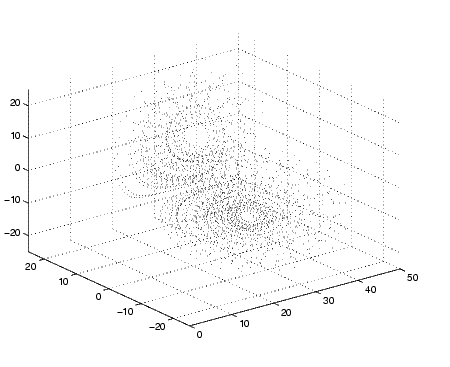GraphicsErase Modes

You can select the method MATLAB uses to redraw graphics objects. One event that causes MATLAB to redraw an object is changing the properties of that object. You can take advantage of this behavior to create animated sequences. A typical scenario is to draw a graphics object, then change its position by respecifying the x-, y,- and z-coordinate data by a small amount with each pass through a loop.

You can create different effects by selecting different erase modes. This section illustrates how to use the three modes that are useful for dynamic redrawing:

• `none`--MATLAB does not erase the objects when it is moved.
• `background`--MATLAB erases the object by redrawing it in the background color. This mode erases the object and anything below it (such as grid lines).
• `xor`--This mode erases only the object and is usually used for animation.

All three modes are faster (albeit less accurate) than the normal mode used by MATLAB.

Example -- Animating with Erase Modes

It is often interesting and informative to see 3-D trajectories develop in time. This example involves chaotic motion described by a nonlinear differential equation known as the Lorenz strange attractor. It can be written

in the formwith a vector-valued function y(t) and a matrix A that depends upon y.The solution orbits about two different attractive points without settling into a steady orbit about either. This example approximates the solution with the simplest possible numerical method -- Euler's method with fixed step size. The result is not very accurate, but it has the same qualitative behavior as other methods.

• ```A = [ -8/3 0 0; 0 -10 10; 0 28 -1 ];
y = [35 -10 -7]';
h = 0.01;
p = plot3(y(1),y(2),y(3),'.', ...
'EraseMode','none','MarkerSize',5); % Set EraseMode to none
axis([0 50 -25 25 -25 25])
hold on
for i=1:4000
A(1,3) = y(2);
A(3,1) = -y(2);
ydot = A*y;
y = y + h*ydot;
% Change coordinates
set(p,'XData',y(1),'YData',y(2),'ZData',y(3))
drawnow
i=i+1;
end
```

The `plot3` statement sets `EraseMode` to `none`, indicating that the points already plotted should not be erased when the plot is redrawn. In addition, the handle of the plot object is saved. Within the `for` loop, a `set` statement references the plot object and changes its internally stored coordinates for the new location. While this manual cannot show the dynamically evolving output, this picture shows a snapshot.Note that, as far as MATLAB is concerned, the graph created by this example contains only one dot. What you see on the screen are remnants of previous plots that MATLAB has been instructed not to erase. The only way to print this graph from MATLAB is with a screen capture.

Background Erase Mode.   To see the effect of `EraseMode` `background`, add these statements to the previous program.

• ```p = plot3(y(1),y(2),y(3),'square', ...
'EraseMode','background','MarkerSize',10,...
'MarkerEdgeColor',[1 .7 .7],'MarkerFaceColor',[1 .7 .7]);

for i=1:4000
A(1,3) = y(2);
A(3,1) = -y(2);
ydot = A*y;
y = y + h*ydot;
set(p,'XData',y(1),'YData',y(2),'ZData',y(3))
drawnow
i=i+1;
end
hold off
```

Since `hold` is still `on`, this code erases the previously created graph by setting the `EraseMode` property to `background` and changing the marker to a "pink eraser" (a square marker colored pink).

Xor Erase Mode.   If you change the `EraseMode` of the first `plot3` statement from `none` to `xor`, you will see a moving dot (`Marker` `'.'`) only. `Xor` mode is used to create animations where you do not want to leave remnants of previous graphics on the screen.

Additional Examples

The MATLAB demo `lorenz` provides a more accurate numerical approximation and a more elaborate display of the Lorenz strange attractor example. Other MATLAB demos illustrate animation techniques.Example -- Visualizing an FFT as a Movie Displaying Bit-Mapped Images© 1994-2005 The MathWorks, Inc.